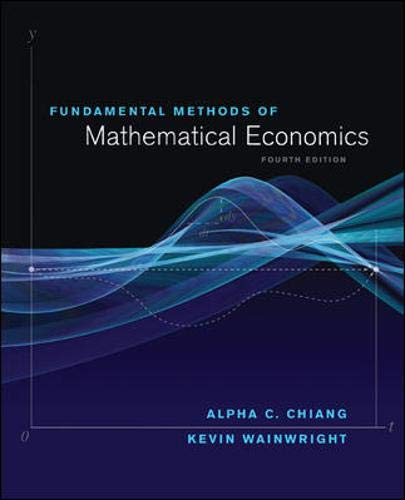Reddit reviews Fundamental Methods of Mathematical Economics

We found 7 Reddit comments about Fundamental Methods of Mathematical Economics. Here are the top ones, ranked by their Reddit score.

Economics
BooksCheck price on Amazon

Math econ isn't a sub-field of, it's a set of mathematical tools used in econ. You can't specialize in math econ in grad school.

Chiang and Wainright and Simon and Blume are what you want to at least cover the basics. Although most if not all of it will be review which is the intention.

The only possible issue I see is your selection of textbook: Principles of Mathematical Economics - I've honestly never heard of this book.

The graduate school go-to textbook is Mathematics for Economists by Simon and Blume. However, I think this book would be overkill for you, as it is geared towards pure, PhD level, economics. Also, I was in a similar place to you, with respect to mathematical training at one point, and Simon &amp; Blume proved to be too large a leap.

My advice would be to use one of the following books (in order of my preference):
1. Essential Mathematics for Economic Analysis by Sydsaeter
2. Mathematics for Economics
by Hoy
3. Fundamental Methods of Mathematical Economics
by Chiang

They'll bring your basic command, of the basic required mathematics up to scratch AND these books cover linear algebra. You will also then be in a good place to tackle Simon &amp; Blume if you ever need to in the future. Another piece of advice: PRACTISE PRACTISE PRACTISE. For what you are doing, you don't need to have a deep understanding of the mathematics you are using BUT, you do need to be very comfortable with applying the techniques.

So, as you are working through (for instance) Sydsaeter, I would be attempting the related practice questions you find in:

1. Schaum's Outline of Calculus
2. Schaum's Outline of Linear Algebra
3. Schaum's Outline of Introduction to Mathematical Economics

Hope this helps.

P.S. Almost all of these books are available for 'free' on Library Gensis

Freedman, Pisani, and Purves is the text I have used for teaching undergrads. Maybe start there for a refresher on probability theory, then move to Degroot and Schervish after if you have time/desire. FPP is very basic and mostly intuition, then DS gets more technical.

You should also brush up on calculus. At least be able to take (and understand) first and second partials. This will save you a lot of trouble later. Then work on linear algebra. Although you’re a psych person, a very good text that will give the basics of both calculus and linear algebra is Chiang and Wainwright, a math Econ book. Despite the difference in substance, it’s the most lucid as well as basic of texts I know of on the subject. So if you’ve never done calculus or linear algebra, this is a good place to start. Chapters 4-7 for basics, 8-10 for more fun.

https://www.amazon.com/Statistics-4th-David-Freedman/dp/0393929728

https://www.amazon.com/Probability-Statistics-4th-Morris-DeGroot/dp/0321500466

https://www.amazon.com/Fundamental-Mathematical-Economics-Wainwright-Professor/dp/0070109109

You can definitely find used, older editions, and international versions that are relatively cheap.

u/504aldo · 2 pointsr/Entrepreneur

not 100% business, but it is still one of my favs. Fundamental Methods of Mathematical Economics

Also, in my wish list:

This. Chiang is the easiest to read and comprehend, while Simon and Blume go into the technical aspects with more proofs and rigor. I took the graduate level mathematical economics as an undergrad and we used Chiang. As a PhD student now, we use Simon and Blume; although I certainly reference Chiang a lot.

Chaing : http://www.amazon.com/Fundamental-Methods-Mathematical-Economics-Wainwright/dp/0070109109/ref=sr_1_1?ie=UTF8&amp;amp;qid=1382493346&amp;amp;sr=8-1&amp;amp;keywords=chiang+economics

S&amp; B : http://www.amazon.com/Mathematics-Economists-Carl-P-Simon/dp/0393957330/ref=sr_1_1?ie=UTF8&amp;amp;qid=1382493357&amp;amp;sr=8-1&amp;amp;keywords=simon+and+blume